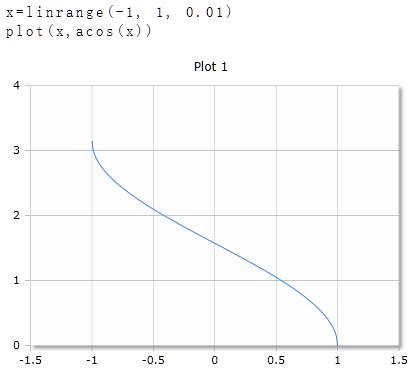# ACos Function

Calculation of the inverse cosine

## Description

The Function $$ACos$$ calculates the inverse cosine of real or complex numbers. The argument can be a single number or a data field. For data fields, the inverse cosine of each element is calculated and the results are returned in a data field of the same size.

## ACos for real numbers

### Parameter

The value of the argument must be between -1 and +1

### Result

The result is given in degrees (full circle = 360°) or radians (full circle = 2 * π). The unit of measurement is set in the toolbar with the DEG or RAD buttons. The Setting applies to the entire worksheet.

If the value of the parameter is outside the range above, the result is a complex number

### Optionaler Parameter

Optionally, a second parameter with the keywords DEG or RAD can be specified to set the unit of measure for this function call. The specification of the parameter has priority over the global setting in the toolbar. For different functions, you can use different units of measurement regardless of the default setting in the toolbar.

ACos (x)

ACos (x, deg)

### Example## ACos for complex numbers

For complex numbers, the argument and the result is always given as radian, regardless of the default setting in the toolbar. The result is also a complex number.

ACos(x + i)

### Example

ACos(2+3i)=1.00-1.98i